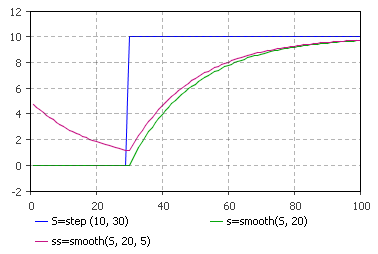AnyLogic

# smooth

smooth is the system dynamics function that returns an exponential smooth of the input.

The plot below illustrates how the function works:smooth can be called in formulas of system dynamics variables and has two notations:

• smooth(input, delayTime, initialValue)

input can be a flow variable, or a numeric expression of any complexity.

delayTime can be either a constant or a numeric expression (e.g. a function call, or a numeric parameter). It should return a positive number: smooth function with zero or negative delay time returns not a number.

• smooth(input, delayTime)

The simplified notation of the function. Is used when the initial value is zero.

#### Units

input — unit

delayTime — time

initialValue — unit

trend() — unit

The output units are the same as the input ones.